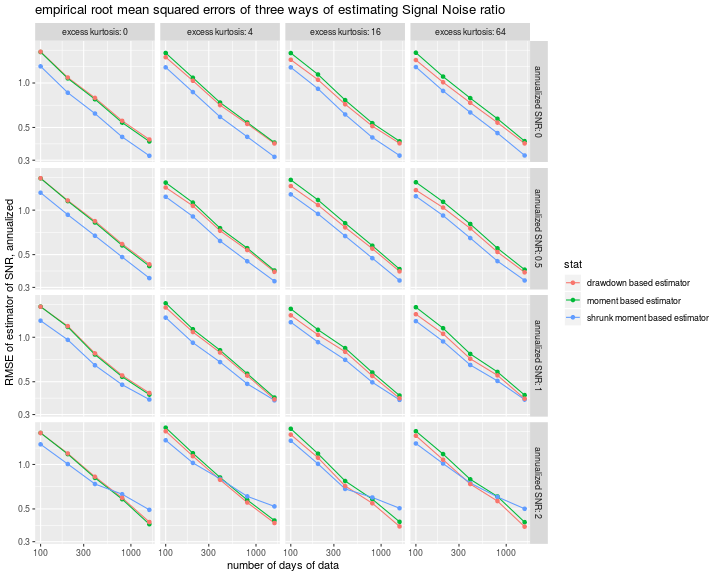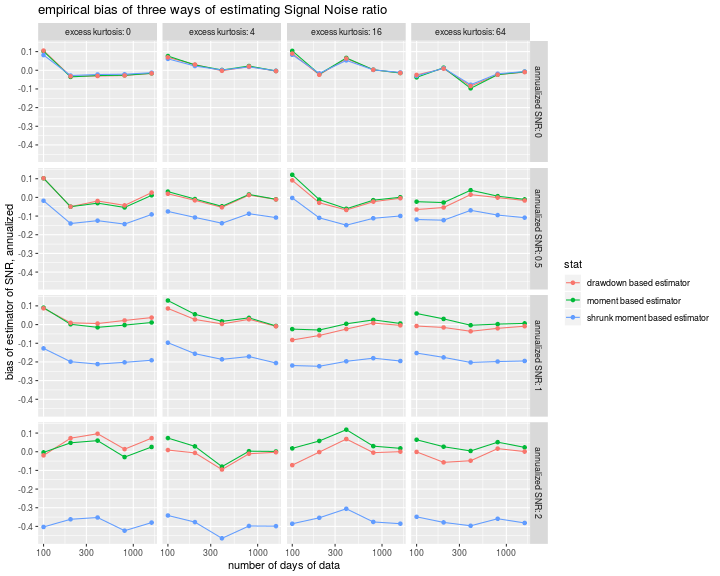# Sharpe Ratio

## A Sharper Sharpe: Just Shrink it!

In a series of blog posts we have looked at Damien Challet's 'Sharper estimator' of the Signal-Noise Ratio, finding it to have lower mean square error than the Sharpe ratio (which we are also calling the 'moment based estimator') for symmetric heavy tailed and skewed returns distributions; in a later post, we compared it to two other estimators for the case of $$t$$-distributed returns. In that last post we noticed that for a substantially easier problem (estimating the mean of a shifted, rescaled $$t$$ when the degrees of freedom and rescaling factor are known), the drawdown estimator seems to achieve lower mean square error than the Cramér Rao lower bound.

From this puzzling result, I suspected that the drawdown estimator is performing some kind of subconcious shrinkage. That is, the drawdown estimator estimates Signal Noise ratio by computing the average, under multiple permutations, number of drawdown and drawup records, then feeds these into a spline function which was built on simulations to map back to Signal Noise ratio. The problem is this reverse mapping may have been built on a limited parameter set with, say, a least squares fit. Values outside of the training range will be pulled back into the training range.

To be concrete, if the training simulations only included Signal Noise ratios between $$-1.5$$ and $$1.5$$ annualized, say, and the process were tested on returns with a Signal Noise ratio of $$3.5$$, say (these are comically large values for illustration purposes), then the spline function would very likely suggest the value is $$1.5$$. While this seems like a catastrope in our hypothetical situation, if you happen to test the estimator on populations which are within the range of Signal Noise ratios used in calibration, you are likely to see reduced mean square error, as we have in our simulations.

Rather than advocate for or against this particular choice of bias-variance tradeoff, here we consider an alternative shrinkage estimator: we take the vanilla Sharpe ratio and multiply it by 0.80. Here we test this basic shrinkage estimator against the Sharpe ratio and the drawdown estimator over a range of sample sizes, Signal Noise ratios, and kurtosis factor for returns drawn from normal or $$t$$ distributions, as in our first study. We compute the empirical root mean square error of each estimator along with the empirical bias over 500 simulations for each parameter setting.

suppressMessages({
library(dplyr)
library(tidyr)
library(tibble)
library(sharpeRratio)
# https://cran.r-project.org/web/packages/doFuture/vignettes/doFuture.html
library(doFuture)
registerDoFuture()
plan(multiprocess)
})

onesim <- function(n,pzeta,gen=rnorm,...) {
x <- gen(n) + pzeta
mux <- mean(x)
sdx <- sd(x)
mome <- (mux + (pzeta - pzeta)) / sdx
sim$SNR })) cbind(pzeta,mome,ddsr) } repsim <- function(nrep,n,pzeta,gen=rnorm) { dummy <- invisible(capture.output(jumble <- replicate(nrep,onesim(n=n,pzeta=pzeta,gen=gen)),file='/dev/null')) retv <- aperm(jumble,c(1,3,2)) dim(retv) <- c(nrep * length(pzeta),dim(jumble)) colnames(retv) <- colnames(jumble) invisible(as.data.frame(retv)) } manysim <- function(nrep,n,pzeta,ex.kurt=0,nnodes=5) { stopifnot(ex.kurt >= 0) # not yet if (ex.kurt==0) { gen <- rnorm } else { thedf <- 4 + 6 / ex.kurt rescal <- sqrt((thedf - 2)/thedf) gen <- function(n) { rescal * rt(n,df=thedf) } } if (nrep > 2*nnodes) { # do in parallel. nper <- table(1 + ((0:(nrep-1) %% nnodes))) retv <- foreach(i=1:nnodes,.export = c('n','pzeta','gen','onesim','repsim')) %dopar% { repsim(nrep=nper[i],n=n,pzeta=pzeta,gen=gen) } %>% bind_rows() } else { retv <- repsim(nrep=nrep,n=n,pzeta=pzeta,gen=gen) } retv } ope <- 252 pzetasq <- c(0,1/4,1,4) / ope pzeta <- sqrt(pzetasq) params <- tidyr::crossing(tibble::tribble(~n,100,200,400,800,1600), tibble::tribble(~kurty,0,4,16,64), tibble::tibble(pzeta=pzeta)) # run it nrep <- 500 set.seed(1234) system.time({ results <- params %>% group_by(n,kurty,pzeta) %>% summarize(sims=list(manysim(nrep=nrep,nnodes=6, pzeta=pzeta,n=n,ex.kurt=kurty))) %>% ungroup() %>% tidyr::unnest() })   user system elapsed 12503.75 4537.97 2532.52  Now I collect the results of the simulation, computing the bias, standard error and root mean square error of the two methods of computing Sharpe. # summarize the moments shrinkage_amt <- 0.80 sumres <- results %>% mutate(shrn=shrinkage_amt * mome) %>% # "shrinkage" group_by(pzeta,n,kurty) %>% summarize(bias_mome=mean(mome - pzeta,na.rm=TRUE), rmse_mome=sqrt(mean((mome - pzeta)^2,na.rm=TRUE)), bias_shrn=mean(shrn - pzeta,na.rm=TRUE), rmse_shrn=sqrt(mean((shrn - pzeta)^2,na.rm=TRUE)), bias_ddsr=mean(ddsr - pzeta,na.rm=TRUE), rmse_ddsr=sqrt(mean((ddsr - pzeta)^2,na.rm=TRUE))) %>% ungroup() %>% arrange(pzeta,n,kurty) %>% tidyr::gather(key=series,value=value,matches('_(mome|ddsr|shrn)$')) %>%
tidyr::separate(series,into=c('metric','stat')) %>%
mutate(stat=case_when(.$stat=='mome' ~ 'moment based estimator', .$stat=='ddsr' ~ 'drawdown based estimator',
.\$stat=='shrn' ~ 'shrunk moment based estimator',
mutate(annualized SNR=signif(pzeta * sqrt(ope),digits=2)) %>%
rename(excess kurtosis=kurty)


Here we plot the RMSE versus sample size. The new shrinkage moment based estimator, plotted in blue, appears to achieve about the same mean square error than the drawdown based estimator, sometimes a little lower, sometimes a little higher.

# plot
library(ggplot2)
ph <- sumres %>%
filter(metric=='rmse') %>%
mutate(value=sqrt(ope) * value) %>% # annualized
ggplot(aes(n,value,color=stat)) +
geom_line() + geom_point() +
scale_x_log10() +
scale_y_log10() +
facet_grid(annualized SNR~excess kurtosis,labeller=label_both) +
labs(y='RMSE of estimator of SNR, annualized',
x='number of days of data',
title='empirical root mean squared errors of three ways of estimating Signal Noise ratio')
print(ph)Here we plot the bias (in annualized units) of the three estimators. The traditional Sharpe ratio is the only estimator among the three that appears to be nearly unbiased. The drawdown estimator is nearly unbiased for the case of normal returns, but has about the same sizable bias for $$t$$ returns.

# plot
library(ggplot2)
ph <- sumres %>%
filter(metric=='bias') %>%
mutate(value=sqrt(ope) * value) %>% # annualized
ggplot(aes(n,value,color=stat)) +
geom_line() + geom_point() +
scale_x_log10() +
facet_grid(annualized SNR~excess kurtosis,labeller=label_both) +
labs(y='bias of estimator of SNR, annualized',
x='number of days of data',
title='empirical bias of three ways of estimating Signal Noise ratio')
print(ph)We are not suggesting that quant managers take a 20% haircut off of their Sharpe ratios! (Although perhaps that's reasonable for backtests.) The point is that a very simple, and truly repugnant, modification to the Sharpe ratio can achieve the same empirical performance as the drawdown estimator. At the same time, the latter lacks any theoretical justification for improved efficiency. Together these cast serious doubts on the drawdown estimator for practical use.